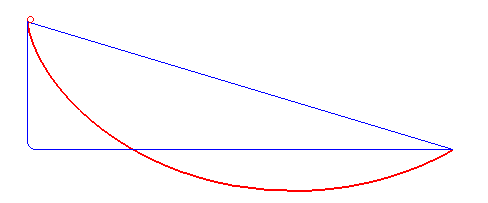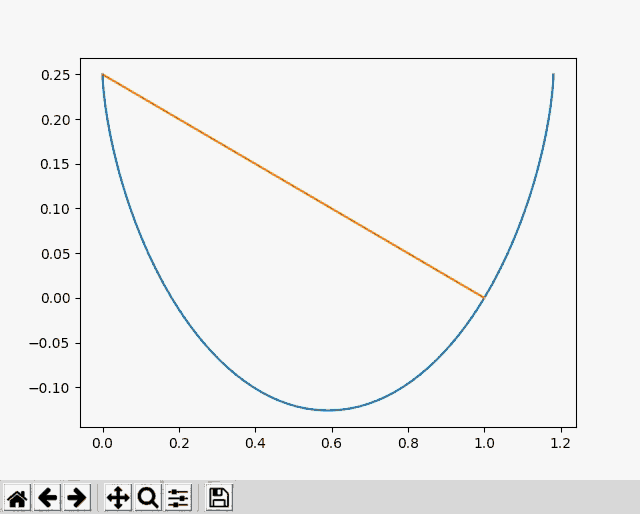# Solving the Brachistochrone with PyTorch

The brachistochrone problem is simple to explain. Imagine a bead which is free to slide on a wire. What shape of wire will get the bead from point A to point B in the shortest time? If you're wondering about the name, "brachistochrone" apparently means "shortest time" in Ancient Greek. Makes sense. The image below shows the optimal path in red, along with some slower paths in blue.This was inspired by Declan, who was using this problem to learn about genetic algorithms. To quote him, "The genetic optimization algorithm may be the greatest concept to ever emerge from the mind of man." A bit dramatic, I think.

The problem can be solved with calculus, but I decided to approach this problem with gradient descent using PyTorch. It seemed like a natural solution, and a good way to learn about Torch. The image below shows my optimization approaching the shape of the known cycloid solution in blue.I divided the wire into segments with heights $$y_i$$ and calculated the speed at each segment using the height difference from the starting point. Then I found the length of the segments using the height differences between points. I calculated the time to reach each point, $$t_i$$, by integrating the segment length divided by the speeds. Finally, I used automatic differentiation to find the derivatives $$\frac{dt_i}{dy_j}$$ and used gradient descent to minimize the time to reach the final point, $$t_{last}$$.